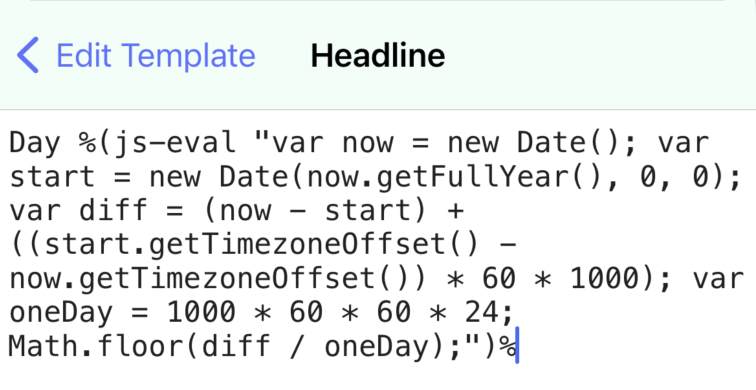Start a new topic

## help with template date macro

Hi. Is there a way to insert the day of the year using the macros in templates?

Yes, but its not obvious!

There isn't currently a macro, such as %year%, which returns the day of the year.

You can however use Scheme in macros. The Scheme in beorg is written in JavaScript, and the simplest way to get the day of the year is to bridge to actually write this in JavaScript.

%(js-eval "var now = new Date(); var start = new Date(now.getFullYear(), 0, 0); var diff = (now - start) + ((start.getTimezoneOffset() - now.getTimezoneOffset()) * 60 * 1000); var oneDay = 1000 * 60 * 60 * 24; Math.floor(diff / oneDay);")%

...where you want the day of the year to appear

In the screenshot below, I have a template where I want the headline to be, for example on 3rd March 2023, "Day 62":Sorry this isn't simpler, but it is possible. To make this easier in practice you can define a Scheme function. For example:

`(define (day-of-year) (js-eval "var now = new Date(); var start = new Date(now.getFullYear(), 0, 0); var diff = (now - start) + ((start.getTimezoneOffset() - now.getTimezoneOffset()) * 60 * 1000); var oneDay = 1000 * 60 * 60 * 24; Math.floor(diff / oneDay);"))`

You can then use the macro as %(day-of-year)%. The above Scheme function can be added to your init.org (see https://beorg.app/learning/initorg/ if you are new to these)

Thank you!!! This actually quite awesome. I’m far from being a programmer by any stretch but just to make sure I am understanding the process, I used a popular generative AI tool to write the javascript portion, to output the day of year as 3digits. Not sure on its correctness, but seems to be working: %(js-eval "var now = new Date(); var start = new Date(now.getFullYear(), 0, 0); var diff = (now - start) + ((start.getTimezoneOffset() - now.getTimezoneOffset()) * 60 * 1000); var oneDay = 1000 * 60 * 60 * 24; var day = Math.floor(diff / oneDay).toString(); day = (day.length < 2 ? '0' : '') + day; day = (day.length < 3 ? '0' : '') + day;")% Thanks for this great app!